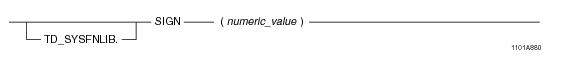# 15.00 - SIGN - Teradata Database

## Teradata Database SQL Functions, Operators, Expressions, and Predicates

Product
Release Number
15.00
Content Type
Programming Reference
Publication ID
B035-1145-015K
Language
English (United States)

## SIGN

### Purpose

Returns the sign of numeric_value.

### Syntaxwhere:

 Syntax element … Specifies … TD_SYSFNLIB the name of the database where the function is located. numeric_value a numeric argument.

### ANSI Compliance

This is a Teradata extension to the ANSI SQL:2011 standard.

### Invocation

SIGN is an embedded services system function. For information on activating and invoking embedded services functions, see “Embedded Services System Functions” on page 24.

### Argument Types and Rules

Expressions passed to this function must have one of the following data types:

BYTEINT, SMALLINT, INTEGER, BIGINT, DECIMAL/NUMERIC, FLOAT/REAL/DOUBLE PRECISION, or NUMBER

### Result Type

The result data type is NUMBER.

For information on the default data type format for NUMBER, see SQL Data Types and Literals.

### Usage Notes

For all numeric types except FLOAT/REAL/DOUBLE PRECISION, SIGN will return the following:

• If numeric_value is < 0, -1 is returned.
• If numeric_value is = 0, 0 is returned.
• If numeric_value is > 0, 1 is returned.
• For FLOAT/REAL/DOUBLE PRECISION, SIGN will return the following:

• If numeric_value is < 0, -1 is returned.
• If numeric_value is >= 0, 1 is returned.
• If the input argument is NULL, the function returns NULL.

### Example

The following query returns the result -1.

`   SELECT SIGN(-2);`

### Example

The following query returns the result 0 since the value is an integer and equal to 0.

`   SELECT SIGN(CAST(0 AS INTEGER));`

### Example

The following query returns the result 1 since the data type is FLOAT and the value is >= 0.

`   SELECT SIGN(CAST(0 as FLOAT));`

### Example

The following query returns the result 1.

`   SELECT SIGN(3.74);`